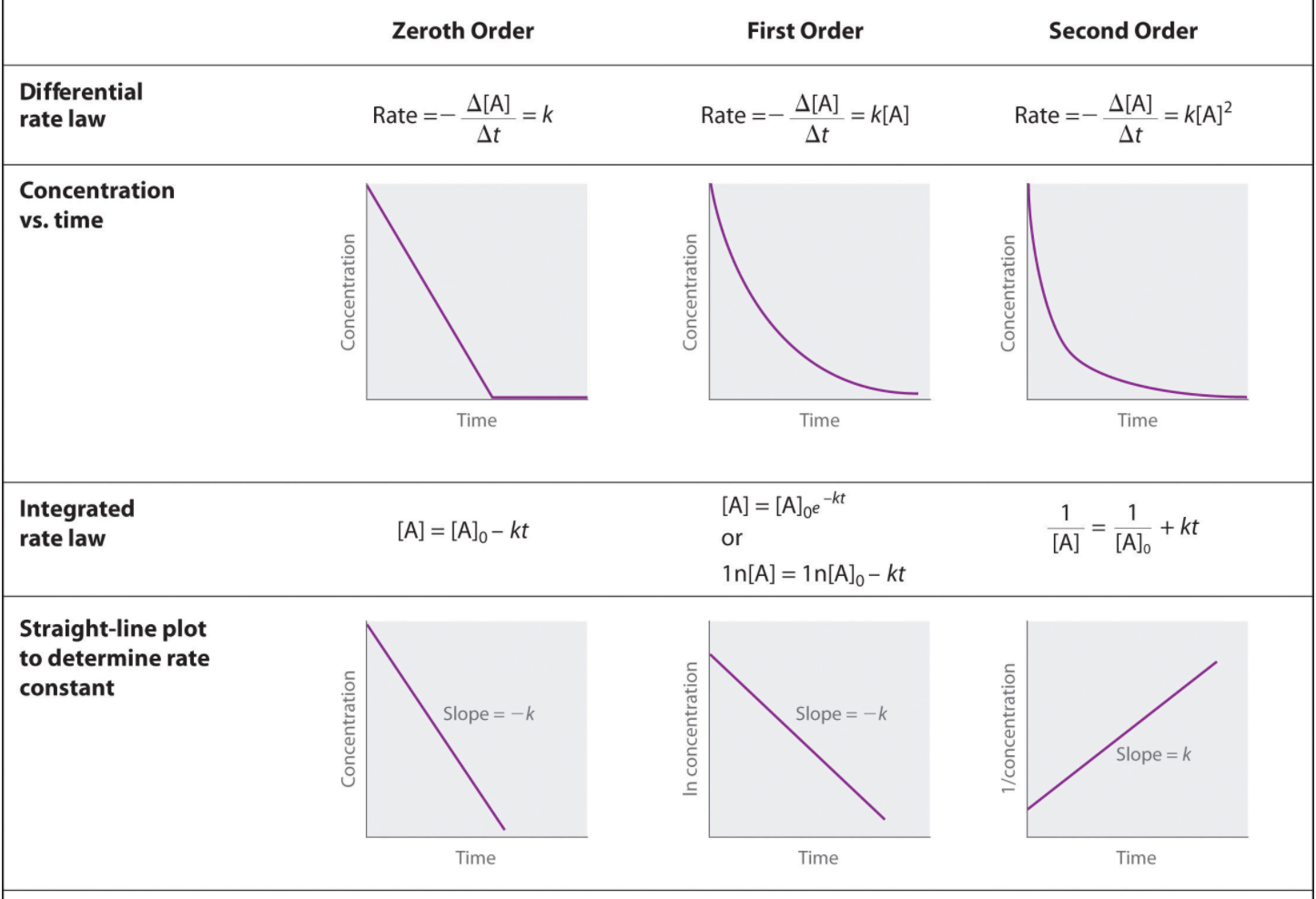# Problem: Please show work and explain:A student generates a plot of ln(k) vs ln[OH-].The result yields a straight line with the equation: y = 1.01x + -0.92According to this data, what is the order of the reaction in OH-, to the nearest whole number?

###### FREE Expert Solution

Recall:We are given: y = 1.01x + -0.92

85% (296 ratings)